##### TI-84 Plus CE Graphing Calculator For Dummies, 3rd EditionYour TI-84 Plus calculator has a massive amount of digits arranged in a list, called a random number table, that it uses to generate random numbers.

## Generating random integers

To generate random integer, follow these steps:

1. To activate the randInt Wizard from the Math PROB menu, press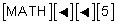If you are using a TI-84 Plus, there is no wizard for the randint command. To use the command, you must know the syntax: randint(lower, upper, [number of elements]).

A wizard makes entering information easy. A wizard arranges the data you enter so that it fits the syntax of the command. See the wizard in the first screen.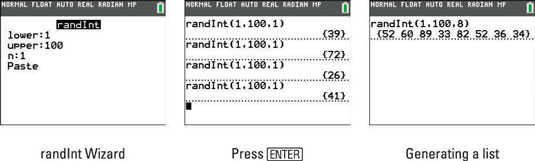2. Enter the lower limit and upper limit you want your random number to be.

You want a random number from 1 to 100. Press [ENTER] or the down-arrow key to navigate to the next line in the wizard.

3. Enter n, for how many random numbers you want to generate.

Press [ENTER] repeatedly to generate more random numbers as illustrated in the second screen.

4. If you want to generate a list of random integers, change the value of n.

See the third screen in.

## Generating random integers with no repetition

Did you notice the integer, 52, was selected twice in the third screen? If you are generating a list of random integers, you can easily avoid repeats by using a different command. Here are the steps:

1. To activate the randIntNoRep Wizard from the Math PROB menu, press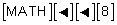See the first screen.

On the TI-84 Plus, the randIntNoRep command does not have a wizard to help you. The syntax for the command is randIntNoRep(lower,upper). Unlike the TI-84 Plus C, you don’t have the option of adjusting the number of terms.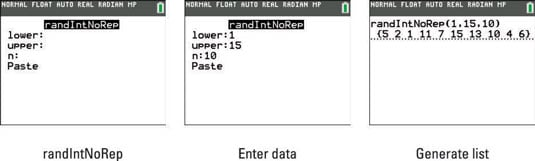2. Enter the upper and lower limits and n (the number of terms).

See the second screen.

3. Press [ENTER] until your numbers have been generated.

This is illustrated in the third screen.

## Generating random decimals

It is easy to generate random decimal numbers that are strictly between 0 and 1. Press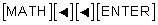to select the rand command from the Math Probability menu. Then repeatedly press [ENTER] to generate the random numbers. The first screen illustrates this process.

To generate random numbers between 0 and 100, use the rand command in an expression: 100*rand. See the second screen.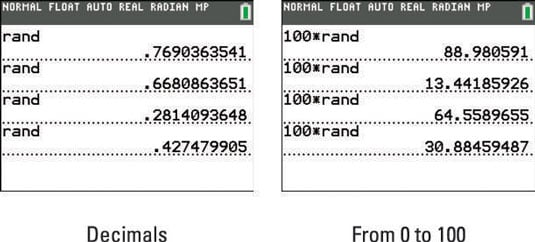## Seeding the random number generator

Your calculator generates random numbers from a massive list of digits arranged in a list. Here is the cool part. You can pick where in the list you want your calculator to start generating random numbers. It is called seeding your random number.

Get a little creative with the number you select to seed your calculator. This should be fun! Figure out how many days you have been alive. Your calculator has a command that can figure that out for you! Follow these steps:

1. Press [2nd] to access the Catalog.

Notice, your calculator is in Alpha mode, indicated by the blinking A in the cursor.

2. To insert the dbd( function, press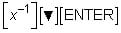dbd stands for days between dates.

3. Enter your birth date as a number in this form: MM.DDYY.

Dates must be between the years 1950 and 2049. Here, June 1, 1968, is entered with the number: 06.0168.

4. Press [,].

5. Enter today’s day as a number in this form: MM.DDYY.

January 2, 2013, is entered with the number: 01.0213.

6. Press [ENTER] to find out how many days you have been alive.

See the first screen. Wow! 16,286 days sounds old!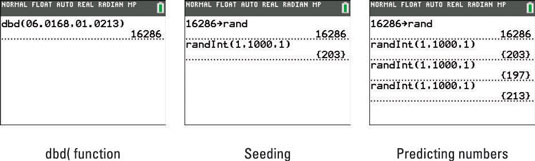Here are the steps to seed your calculator:

1. Enter the number you are using to seed your calculator.

16286. Of course, you could use any real number to seed your calculator.

2. Press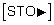3. To insert the rand command, press4. Press [ENTER] to seed your calculator.

See the first line in the second screen.

5. Try it out! Use randInt( to generate a random number.

See the last line in the second screen.

Want to impress your friends? Seed your calculator with results you know in advance. For example, if you secretly seed your calculator with the number 16286, then the next three random numbers (from 0 to 1,000) that will be generated will be 203, 197, and 213, as shown in the third screen. Just don’t share your secret!